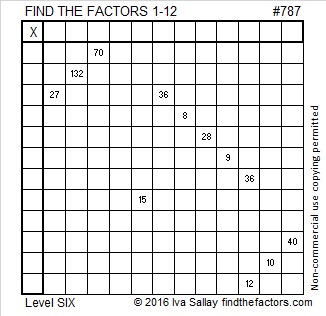# 787 Always a Unique Solution

• 787 is a prime number.
• Prime factorization: 787 is prime.
• The exponent of prime number 787 is 1. Adding 1 to that exponent we get (1 + 1) = 2. Therefore 787 has exactly 2 factors.
• Factors of 787: 1, 787
• Factor pairs: 787 = 1 x 787
• 787 has no square factors that allow its square root to be simplified. √787 ≈ 28.05352.Now for today’s puzzle….The fact that these Find the Factor puzzles always have a unique solution is an important clue in solving this rather difficult puzzle. Good luck!Print the puzzles or type the solution on this excel file: 12-factors-782-787

—————————————–

Here’s more about the number 787:

787 is a palindrome in bases 4, 10, 11 and 16:

• 30103 BASE 4; note that 3(256) + 0(64) + 1(16) + 0(4) + 3(1) = 787
• 787 BASE 10; note that 7(100) + 8(10) + 7(1) = 787
• 656 BASE 11; note that 6(121) + 5(11) + 6(1) = 787
• 313 BASE 16; note that 3(256) + 1(16) + 3(1) = 787

—————————————–

What did I mean when I wrote that the puzzles always having a unique solution is an important clue? There is only one clue in the puzzle that is divisible by 11. One of the rows and one of the columns do not have a clue, so the other 11 will go with one of them. The cell where the empty row and empty column intersect cannot be 132 because if that worked, it would produce two possible solutions to the puzzle. This table explains a logical order to find the solution.